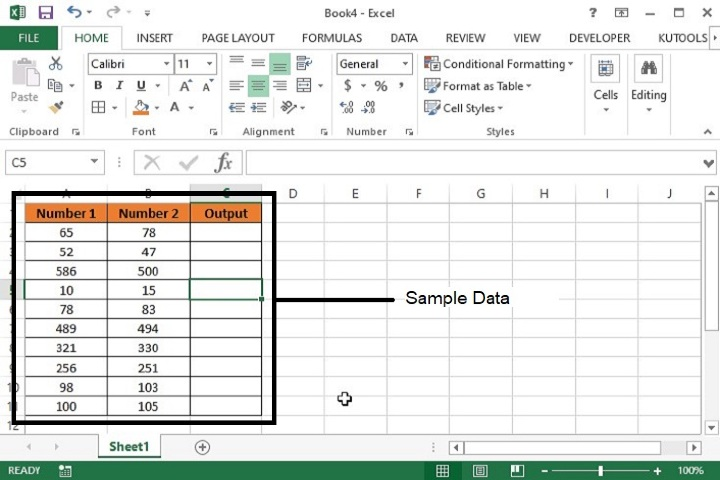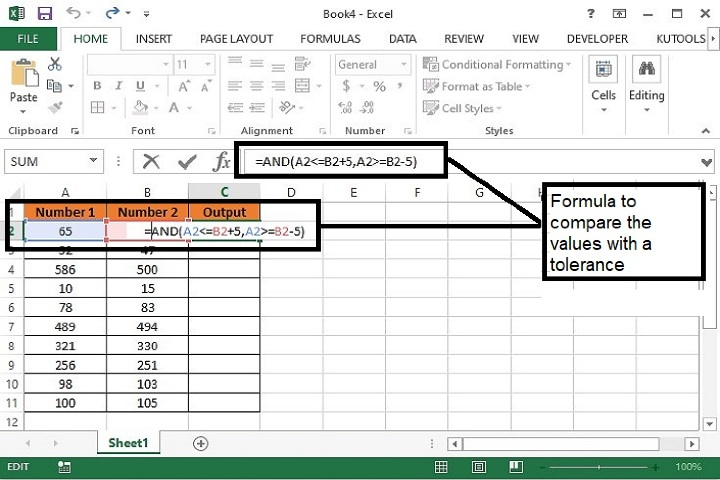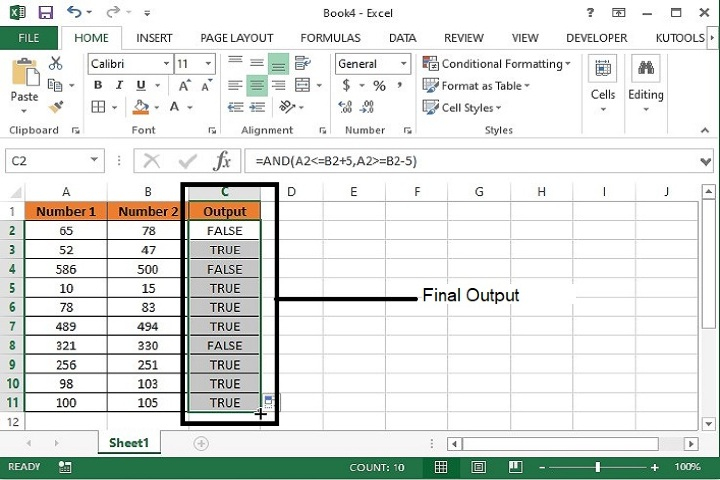# How to compare numbers but allowing a tolerance in Excel?

In this article we will learn how to compare two number having nearby values to each other with a specified tolerance value. However using the basic formula as =A1=B1 we can directly compare the values for the exact match but sometimes we need to compare the value upto a tolerance values. For this, follow the below mentioned formula and steps.

## Comparing Two Numbers having a Specified Tolerance Value

Step 1 − We have taken the following sample data for comparison.Step 2  Enter the formula in C2 cell as mentioned below. This formula will return True for those values where tolerance is equals to 5 or False when tolerance value is greater than 5 or less than 5.

=AND(A2<=B2+5,A2>=B2-5)Step 3  Now, drag the formula till the last row of data and the final output will be as following.Formula Syntax Description

Argument

Description

AND(logical_1, logical_2, logical_3)

AND function returns True if all the conditions are true in logic and returns False if any of the conditions is false.

## Conclusion

Hence using the above formula, adjacent numbers as well as numbers of different cells can be compared with a tolerance value. However, one more formula can also be used for the same activity i.e. =ABS(A2-B2) <=”ToleranceValue”. This will also work in the same way as mentioned above.

Updated on: 02-May-2023

312 Views